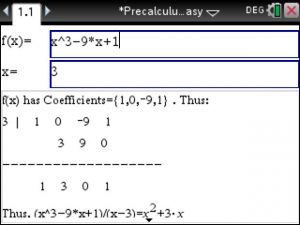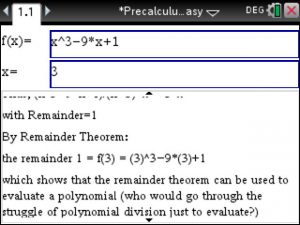## ▷Factor Theorem and Remainder Theorem – Step by Step – using the TiNspire CX – Precalculus Made Easy

In Precalculus, Factor Theorem and Remainder Theorem are very common topics and if you have your TiNspire CX available you can perform both theorems step by step. Just pull out your TiNspire CX CAS and start PreCalculus Made Easy app (Download at www.TiNspireApps.com) and enter Polynomial and the number a.

The Factor Theorem is then performed automatically as shown below:The Remainder Theorem is just straight forward. See below:Posted on Categories preCalculus, tinspirecx